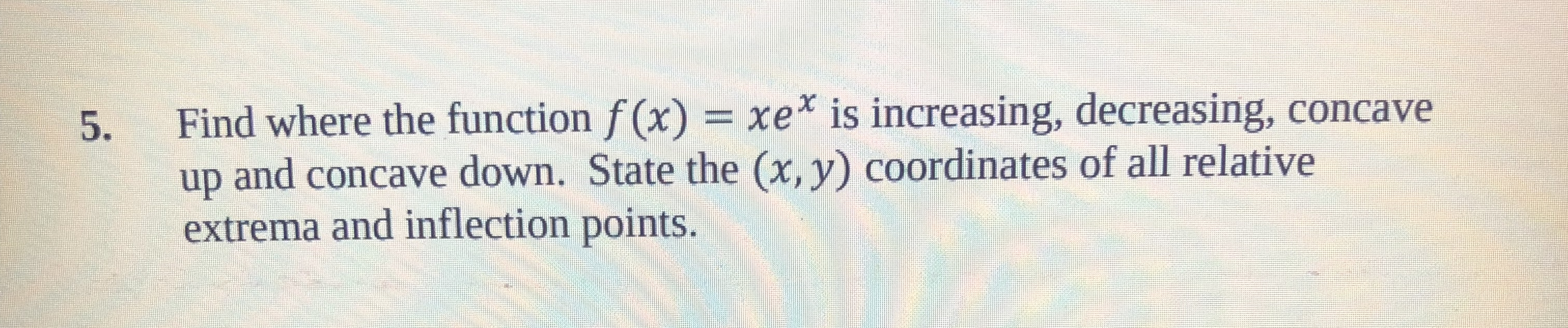5. Find where the function f (x) xe is increasing, decreasing, concaveup and concave down. State the (x, y) coordinates of all relativeextrema and inflection points.

Questionhelp_outlineImage Transcriptionclose5. Find where the function f (x) xe is increasing, decreasing, concave up and concave down. State the (x, y) coordinates of all relative extrema and inflection points. fullscreen
Step 1

Given information:

The given fucntion is f(x)=xe^x.

Step 2

Calculation:

Step 3

f(-1)=-1e^-1

=-1/e

Critical point is (-1,-1/e).

(-infinity, -1)U(-1, +infinity)

Assume x=-2

f(-2)=-2e^(-2)<0

f(2)=2e^2>0

So, the function is inc...

Want to see the full answer?

See Solution

Want to see this answer and more?

Our solutions are written by experts, many with advanced degrees, and available 24/7

See Solution
Tagged in

Calculus